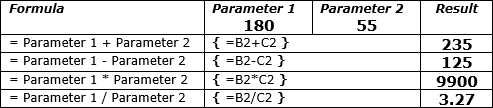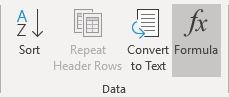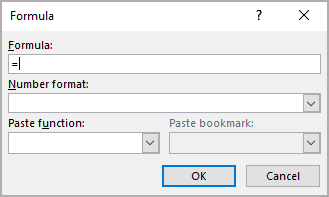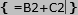# How to add, subtract, multiply and divide cells in a Word table

Word
Most of the people know that Word is for writing texts sometimes containing tables, and Excel is all about tables and calculations for them. But what if you need to create a Word table with formula. Do you need to create a table in Excel and insert it into Word? No, you don't. You can create formulas in Word to perform simple arithmetic calculations, such as addition, subtraction, multiplication, or division.

If you create the table in Excel and paste it into Word document, updates of the table data become difficult because you need to launch embedded Excel for each change. Moreover, the visual appearance of Word and Excel tables is different, and you will need a lot of time on the styling of the Excel table. For some situations, this is not practical because it is faster to insert and change simple formulas in the Word document (see How to reference a cell of a Word table):To add a formula into the Word table (see How to calculate formulas in a Word document if you need to use formulas without tables), do the following:

1.   Position the cursor where you want to paste a formula.

2.   On the Table Layout tab, in the Data group, click the Formula button:3.   In the Formula dialog box, enter the formula:Unfortunately, Word proposes only simple operations and functions that can work without Excel (see Functions and formulas in Word). However, you still can create many simple formulas instead of using the calculator. Cell references in formulas are similar to Excel: A1, B12, etc.

Note: The Formula dialog box is not your only option, you can press Ctrl+F9 to create a field and type a formula inside the field braces:See also this tip in French: Comment ajouter, soustraire, multiplier et diviser des cellules dans un tableau Word.

If you have any questions or suggestions, please feel free to ask OfficeToolTips team.